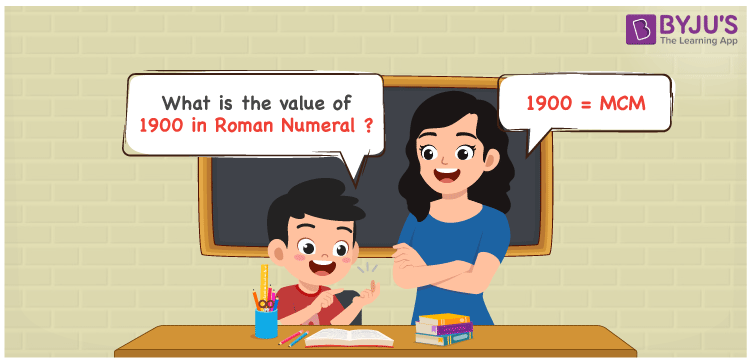# 1900 in Roman Numerals

1900 in Roman Numerals is represented as MCM. The letters used by the ancient Romans to express numbers is known as Roman Numerals. The article  Roman Numerals 1 to 1000 in simple language help students to learn the Roman letters from 1 to 1000 effectively. Let us discuss how to convert 1900 into Roman Numerals with a detailed explanation in this article.

 Number Roman Numeral 1900 MCM

## How to Write 1900 in Roman Numerals?We can express the number 1900 in Roman Numerals by following the method given below

• First, break the number 1900 into the least expandable form
• 1900 = 1000 + (1000 – 100)
• Write their respective Roman letter and add/subtract them
• 1900 = M + (M – C) = MCM
• Hence, the value of 1900 in Roman Numerals is MCM.

## Video Lesson on Roman Numerals## Frequently Asked Questions on 1900 in Roman Numerals

Q1

### What is 1900 in Roman Numerals?

1900 in Roman Numerals is MCM.
Q2

### What is the value of 1000 + 900 in Roman Numerals?

1000 + 900 = 1900. The number 1900 in Roman Numerals is MCM. Hence the value of 1000 + 900 in Roman Numerals is MCM.
Q3

### Find the difference between (20 – 50) + 1900 in Roman Numerals.

(20 – 50) + 1900 = 1870. The number 1870 in Roman Numerals is MDCCCLXX. Thus the difference between (20 – 50) + 1900 in Roman Numerals is MDCCCLXX.